# Time series forecasting with Facebook Prophet

If you are monitoring your web services with Wavefront, Prometheus, Grafana etc. then you are aware of time series. In this post I am sharing my learnings about time series forecasting. Starting with some basics of time series, then using a library from Facebook called Prophet to forecast and finally some thoughts on how this could work in for real world scenarios.

To start here is a sample hourly request data for a hypothetical service for 4 weeks (28 days * 24 = 672 data points). Each row represents average number of calls per second to your server in that hour. I will use this in all the examples.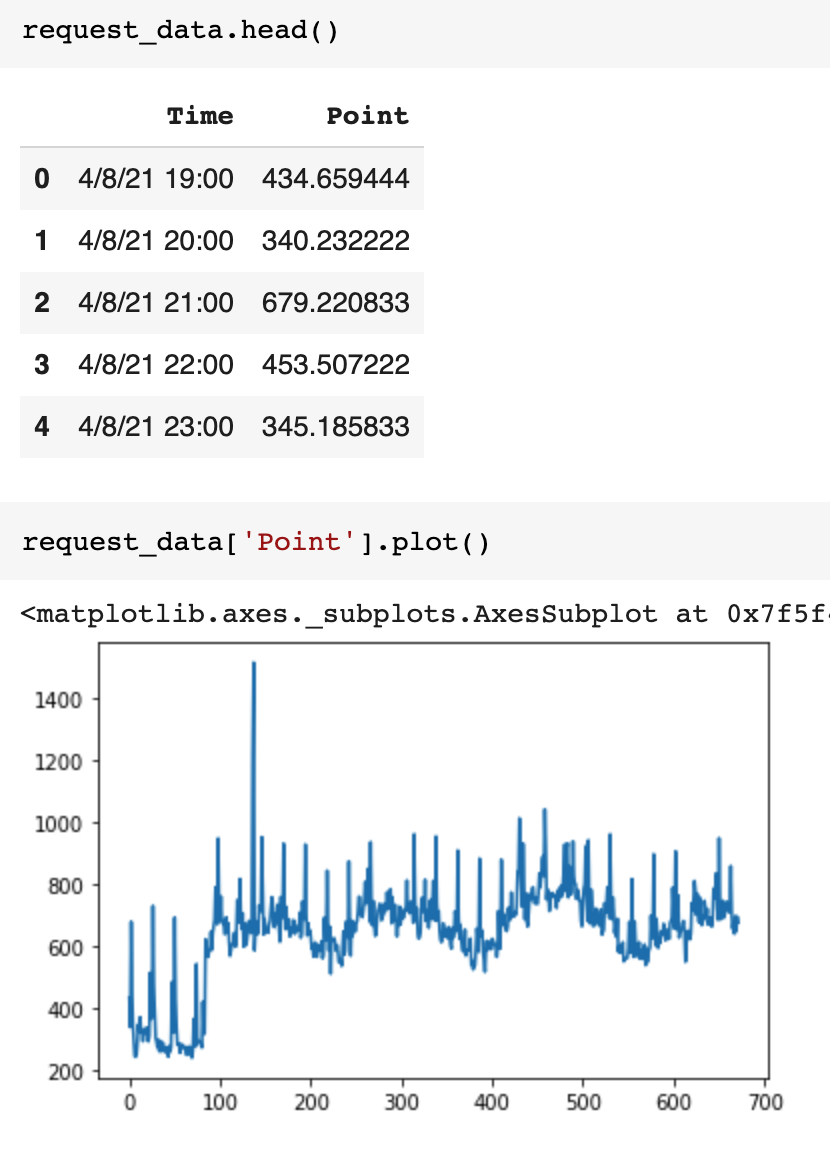Hypothetical request rate data

# Stationarity and Autocorrelation

In a stationary time series, the mean and variance is generally constant over time (reference).

a stationary time series is a series whose statistical properties are independent of the point in time at which they are observed

Intuitively this should make it easy to understand why stationarity helps in more accurate forecasts. There are statistical tests you can apply (beyond my scope) so here is a simple visualization. In general the size of each candle is similar so this could be considered stationary time series (there are some outliers like day 12) (reference).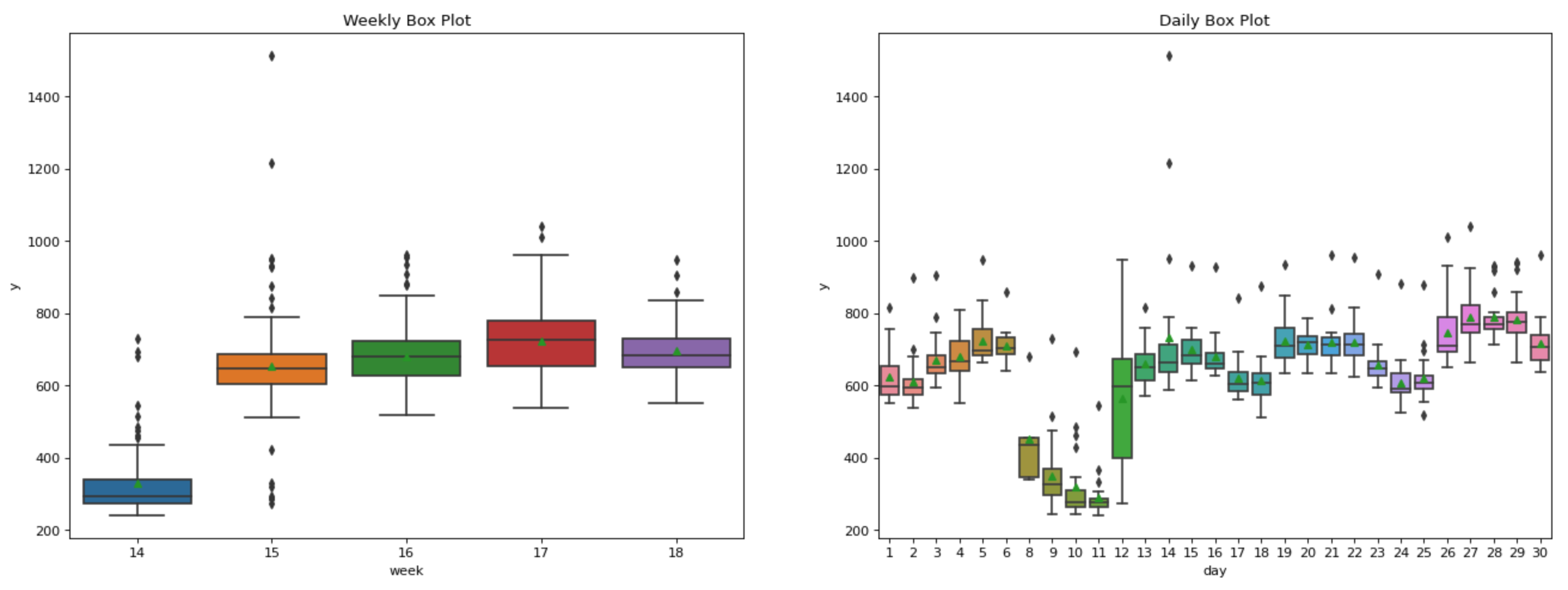Weekly and daily boxplots with mean

Autocorrelation function (ACF) is the degree of similarity of the time series when lagged by itself and represents how similar the current value is to past values. In ACF all correlations between current past are used and these are direct and indirect effects. Additionally, there is another Partial Autocorrelation (PACF) function that represent to how similar the current value is to the past but eliminates all the indirect effects (reference). This specifically applies to autoregressive (AR) models which we will use later. There are other moving average (MA) models as well which are not discussed.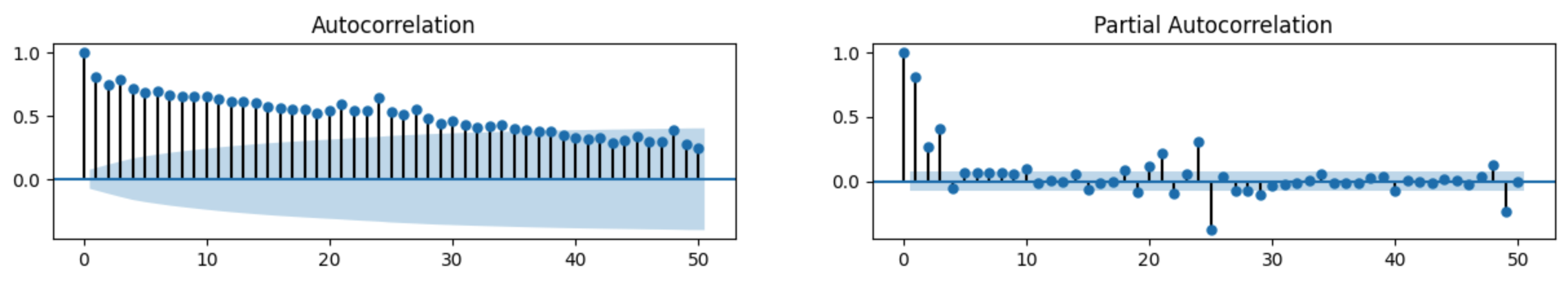Autocorrelation and Partial Autocorrelation

# Time Series Components

1. Trend — over a period of time (x-axis left to right) are the values (y-axis) going up or down or remain the same? For example, there may be ups and downs but generally in the last 20 years the total stock index has an upwards trend.
2. Seasonality — is there some repeating pattern? For example, summer vacations, Thanksgiving and Christmas would have increased number of people traveling. The “seasons” could be within a day, for example a retail ecommerce site may have more traffic during the day and less at night. Holidays — Seasonality maybe impacted by certain special circumstances like a pandemic and these are known as holidays. Again, for a retail web service during the black Friday week there will be a spike that interrupts the usual seasonality. Additionally, we can also consider new feature launch as a seasonal effect.
3. Residual — this is the “noise” and other unexplained component of a time series.

There are 2 ways to decompose a time series into these components: Additive and Multiplicative.

## Additive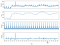Additive decompose of example data

This is roughly represented as:

`y(t) = Trend(t) + Seasonality(t) + Residual(t)`

## Multiplicative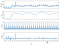Multiplicative decompose of example data

This is roughly represented as:

`y(t) = Trend(t) * Seasonality(t) * Residual(t)`

## Additive vs Multiplicative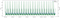Additive and multiplicative seasonality

In the case of web-services traffic I will try both but generally multiplicative is likely the right choice. This is because seasonal variations generally follow the trend and affect the series by a large value.

# Forecasting with Facebook Prophet

`fb = Prophet()fb.fit(request_data)future_dates = fb.make_future_dataframe(periods=7*24, freq='H')forecast = fb.predict(future_dates)fb.plot(forecast);`

Default is additive and I tired with both additive and multiplicative. The `forecast` above contains all the predictions and the upper and lower bounds including those of the components. In my case they appear identical though as discussed above multiplicative is probably the better approach.

The black dots are actual values while the blue line is from the model.

## Additive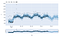Additive forecast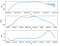Additive components

## Multiplicative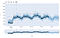Multiplicative forecast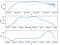Multiplicative components

## Forecast vs Observed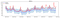Additive forecast vs observed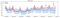Multiplicative forecast vs observed

There are lots of knobs to control and while the Prophet documentation mentions these you actually need to understand a lot of details and understand internals before you can play with them.

All the code and sample data for above is in Github at this location.

# Real World

When collecting metrics, each metric can have N arbitrary key-value labels (see Prometheus example) so when considering such time series we need to extract series based on some label combinations or apply additional regressors or potentially a both? Further forecasts created would then need to be recombined in some way?

Another problem is scaling. If the time series is specific to a specific customer (for example forecasting use for a single customer), then the model trained cannot be applied to all customers and each must be individually trained? Components such as seasonality may apply across customers and that maybe applicable to all?

Finally building and operating data pipelines is something I have no knowledge about so lots of new things to learn!

If these topics interest you then reach out to me, and I will appreciate any feedback. If you would like to work on such problems you will generally find open roles as well! Please refer to LinkedIn.

## More from Rahul Agarwal

https://linkedin.com/in/rahulaga